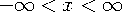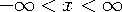Functions and CALL Routines

# ARSINH Function

Returns the inverse hyperbolic sine.
 Category: Hyperbolic

## Syntax

 ARSINH(x)

### Arguments

x

specifies a numeric constant, variable, or expression.

 Range:The ARSINH function computes the inverse hyperbolic sine. The ARSINH function is mathematically defined by the following equation, where:Replace the infinity symbol with the largest double precision number that is available on your machine.

The following example computes the inverse hyperbolic sine.

```data _null_;
x=arsinh(5);
x1=arsinh(-5);
put x=;
put x1=;
run;```

SAS writes the following output to the log:

```x=2.3124383413
x1=-2.312438341```

 Functions:Previous Page | Next Page | Top of Page